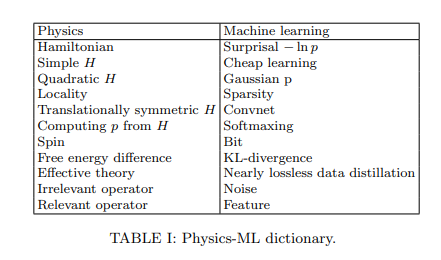# Why Does Deep and Cheap Learning Work So Well?

Source: Deep Learning on Medium# Why Does Deep and Cheap Learning Work So Well?

This papers talks about the effectiveness of Deep Learning. Particularly the paper raises the question Why does deep learning works so well ? Not only deep learning is good but it does that with extremely small number of parameters compared to the huge space of all possible input combinations? . The authors refer this as “Cheap Learning”. We will try to answer some of those questions later in this post.

This paper focuses mainly on expressibility and efficiency of Deep NNs. What do these terms mean? The paper discusses these and other factors to decide the quality of any machine learning model :

• Expressibility: What class of functions can the neural network express? We know that even with a neural network with a single hidden layer and non-linearity ( eg. sigmoid) can approximate any function within some defined error ε with some probability p[Cybenko].
• Efficiency: How much resources (neurons, parameters, etc.) does the neural network require to approximate a given function? Again, Cybenko’s paper doesn’t provide the exact number of hidden units required but only says that if you have a large number of hidden units + some activation you can approximate any function.
• Learnability: How rapidly can the neural network learn good parameters for approximating a function? In deep learning people use different optimizers such as SGD and its variants to iteratively find the best parameters. What are the convergence rate of such optimization process for different type of models ?

The million dollar question: “How can neural networks approximate functions well in practice, when the set of possible functions is exponentially larger than the set of practically possible networks?”

For an 8-bit image of size 1000 x 1000 there are 256¹⁰⁰⁰⁰⁰⁰ possible images. Consider a classification task to find p(y|x), where x is the feature and y is the output class. There are a possible 256¹⁰⁰⁰⁰⁰⁰ ways of an image being of a particular category y. To put things into perspective, 256¹⁰⁰⁰⁰⁰⁰ is a very large number, greater than atoms in our universe (about 10⁷⁸).

One really interesting connection that the paper makes is many terms that are already known in physics.